# How to convert time to decimal number, hours, minutes or seconds in Excel

The tutorial demonstrates different ways to convert time to decimal in Excel. You will find a variety of formulas to change time to hours, minutes or seconds as well as convert text to time and vice versa.

Because Microsoft Excel uses a numeric system to store times, you can easily turn hours, minutes and seconds into numbers that you can use in other calculations.

In general, there are two ways to convert time to decimal in Excel - by changing the cell format and by using arithmetic calculations or Excel time functions, such as HOUR, MINUTE and SECOND. Further on in this tutorial, you will find the detailed explanation of the first way and formula examples demonstrating the other technique.

## How to convert time to decimal number in Excel

Overall, there are three ways to change a time value to a decimal number: arithmetic operation, CONVERT function or a combination of three different Time functions.

The easiest way to convert time to decimal in Excel is to multiply the original time value by the number of hours, seconds or minutes in a day:

• To convert time to a number of hours, multiply the time by 24, which is the number of hours in a day.
• To convert time to minutes, multiply the time by 1440, which is the number of minutes in a day (24*60).
• To convert time to seconds, multiply the time time by 86400, which is the number of seconds in a day (24*60*60 ).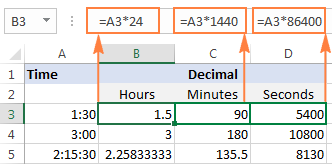In the following sections, you will learn the other methods of converting times to a decimal number in Excel.

### How to convert time to hours in Excel

This section demonstrates 3 different formulas to convert hours from the standard time format (hh:mm:ss) to a decimal number.

#### Formula 1: Arithmetic calculation

You already know the fastest way to convert a time value to a number of hours in Excel - multiplying by 24, i.e. by the number of hours in one day:

`=A2*24` (where A2 is the time value)

To get the number of compete hours, embed the above formula in the INT function that will get rid of the fractional part:

`=INT(A2*24)`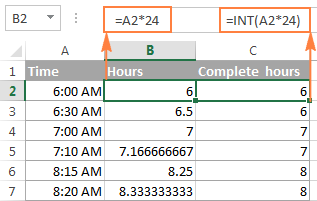#### Formula 2: CONVERT function

Another way to perform the "time > hours" conversion is to use the following Convert formula:

`=CONVERT(A2, "day", "hr")`

#### Formula 3: HOUR, MINUTE and SECOND functions

Finally, you can use a bit more complex formula, whose logic, however, is quite obvious. Extract the individual time units by using the HOUR, MINUTE and SECOND functions, then divide minutes by 60 (the number of minutes in an hour) and seconds by 3600 (the number of seconds in an hour), and add up the results:

`=HOUR(A2) + MINUTE(A2)/60 + SECOND(A2)/3600`

### How to convert time to minutes in Excel

The same three methods can be used to convert minutes from the standard time format to a decimal number.

#### Formula 1: Arithmetic calculation

To convert time to total minutes, you multiply time by 1440, which is the number of minutes in one day (24 hours * 60 minutes = 1440):

`=A2*1440`

If you want to return the number of compete minutes, utilize the INT function like in the previous example:

`=INT(A2*1440)`

You can view the results in the screenshot below: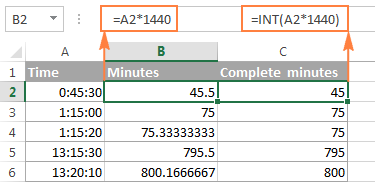#### Formula 2: CONVERT function

To do the "time > minutes" conversion with the CONVERT(number, from_unit, to_unit) function, supply "day" as the unit to convert from and "mn" as the unit to convert to:

`=CONVERT(A2, "day", "mn")`

#### Formula 3: HOUR, MINUTE and SECOND functions

One more way to get the number of minutes is to multiply hours by 60 and divide seconds by the same number:

`=HOUR(A2)*60 + MINUTE(A2) + SECOND(A2)/60`

### How to convert time to seconds in Excel

Converting time to total seconds in Excel can be done in a similar fashion.

#### Formula 1: Arithmetic calculation

Multiply the time value by 86400, which is the number of seconds in a day (24 hours * 60 minutes * 60 seconds = 86400):

`=A2*86400`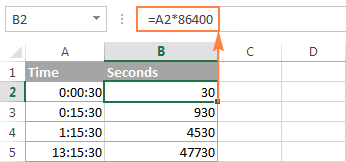#### Formula 2: CONVERT function

The formula is basically the same as in the above examples with the only difference that you convert the "day" unit to "sec":

`=CONVERT(A2, "day", "sec")`

#### Formula 3: HOUR, MINUTE and SECOND functions

Use the HOUR, MINUTE and SECOND functions like in the two previous examples (I believe at this point you don't need any further explanation of the formula's logic :)

`=HOUR(A2)*3600 + MINUTE(A2)*60 + SECOND(A2)`

Tips:

1. If any of the above formulas returns a value formatted as time, simply change the cell's format to Generalto display it as a number.
2. To convert time to a decimal number that represents the time in the internal Excel system, apply the General format to the cell. With this approach, 23:59:59 will be converted to 0.99999, 06:00 AM to 0.25, and 12:00 PM to 0.5. If an integer part of the converted number is greater than zero, it means that your cell contains both date and time values.

## How to split date and time in Excel

As is often the case, your Excel worksheet may contain dates and times in one cell, while you want to split them into two separate cells.

Remembering that in the internal Excel system the date value is stored as a whole part and the time value as a fractional part of a decimal number, you can extract the date using the INT function, which rounds the cell value down to the nearest integer.

Supposing your original dates and times are in column A, the following formula will accomplish the separation:

`=INT(A2)`To extract the time portion, subtract the date returned by the above formula from the original date and time value:

`=A2-B2`

Where column A contains the original date & time values and column B contains the dates returned by the INT function.

If you'd rather not have time values linked to the separated dates (for example, you may want to remove the date column in the future), you can use the following MOD formula that refers to the original data only:

`=MOD(A2,1)`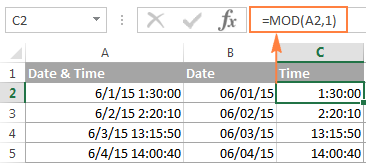Tip. If the separated date and time values are not displayed properly, change the format of the new columns to Date and Time, respectively.

This is how you split date and time in Excel. If you want to further separate hours, minutes and seconds into individual columns, then use the HOUR, MINUTE and SECOND functions, as demonstrated in How to get hours, minutes and seconds from a timestamp.

## How to spell time in Excel

Sometimes, you may need to convert time into the format that reads something like "# days, # hours, # minutes and # seconds". A good thing is that you already know all the ingredients of the formula:

• Extract days using the INT function;
• Extract time units with HOUR, MINUTE and SECOND functions, and
• Concatenate all parts in a single formula.

Having difficulties with figuring out a proper formula for your worksheet? The following example will make things easy!

Supposing you have the dates of upcoming events in column B beginning in cell B4, and the current date and time returned by the NOW() function in cell B1.

The formula to calculate the time difference is as simple as `=B4-\$B\$1`. Of course, nothing prevents you from subtracting the current date and time directly with `=B4-NOW()`.

And now, let's make a countdown timer that would show how many days, hours, minutes and seconds are left until each event.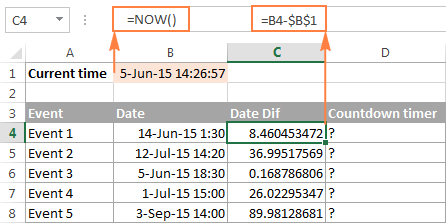The formula to enter in cell D4 is as follows:

`=INT(C4) & " days, " & HOUR(C4) & " hours, " & MINUTE(C4) & " minutes and " & SECOND(C4) & " seconds"`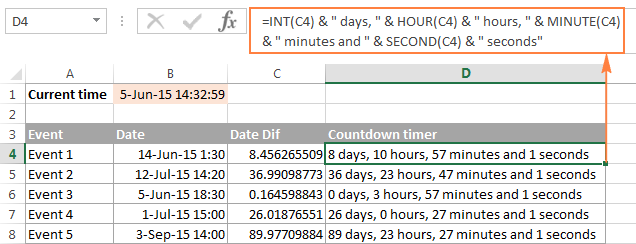If you wish to get rid of 0 values, like in cells D6 and D7 in the screenshot above, then include the following IF statements:

`=IF(INT(C4)>0, INT(C4)&" days, ","") & IF(HOUR(C4)>0, HOUR(C4) & " hours, ","") & IF(MINUTE(C4)>0, MINUTE(C4) & " minutes and ","") & IF(SECOND(C4)>0, SECOND(C4) & " seconds","")`

All zeros are gone!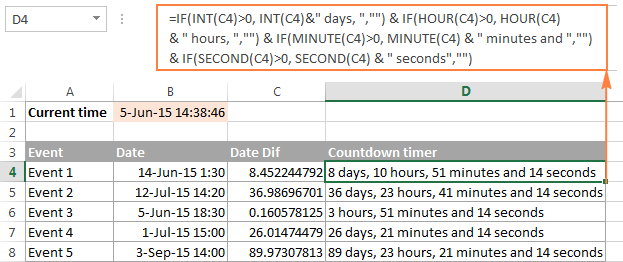Note. When either of the above formulas refers to a negative number, the #NUM! error will appear. This may happen when you subtract a bigger time from a smaller one.

An alternative way to write time in words in Excel is to apply the following custom time format to the cell: d "day," h "hours," m "minutes and" s "seconds". No formulas and no calculations are required! For more information, please see Creating a custom time format in Excel.

## Convert text to time in Excel

If your time formulas and calculations do not work right, time values formatted as text is often the cause. The fastest way to convert text to time in Excel is using the TIMEVALUE function.

The Excel TIMEVALUE function has just one argument:

TIMEVALUE(time_text)

Time_text is a text string in any of the time formats that Excel can recognize. For example:

`=TIMEVALUE("6:20 PM")`

`=TIMEVALUE("6-Jan-2015 6:20 PM")`

`=TIMEVALUE(A2)` where A2 contains a text string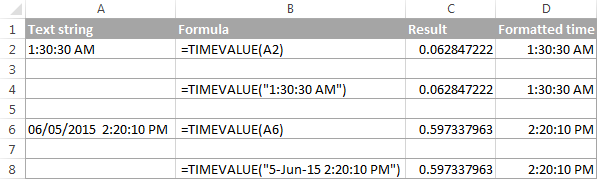As you see, the formulas with cell references and corresponding text strings deliver identical results. Also, please notice the left alignment of time strings (text values) in cells A2 and A6 and right-aligned converted time values in column D.

## Convert time to text in Excel

Supposing you have an Excel file full of times formatted to look like "8:30:00 AM" and you want to convert them to the text format. Simply changing the cell's format to TEXT won't work because this would change your time values to underlying numeric representation of the time. For example, 8:30:00 AM will be converted to decimal 0.354166666666667.

So, how do you convert cells to the text format so that your cells still have the time in them? The answer is to use the TEXT function that converts a numeric value to text with the display formatting that you specify, for example:

`=TEXT(\$A2,"h:mm:ss")`

The screenshot below demonstrates other possible formats: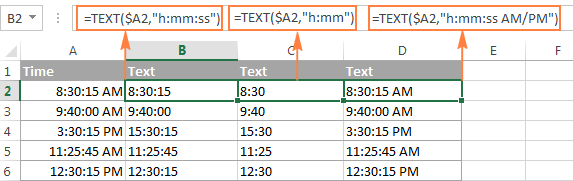## How to convert numbers to time format in Excel

If you have a list of numbers such as 1, 2, 3.5 and you want to convert them to a time format, for example 1:00:00, 2:00:00 or 3:30 AM, perform the following steps.

1. Divide the numbers by 24 (there are 24 hours in a day). The formula can be as simple as =A2/24.
2. Select the cell(s) with the formula result, right-click and select Format Cells from the context menu or press Ctrl+1. Either way, the Format Cells dialog will appear, you select Time on the left pane under Category, and choose the format you want on the right pane under Type. Please see How to apply or change the time format in Excel for more details.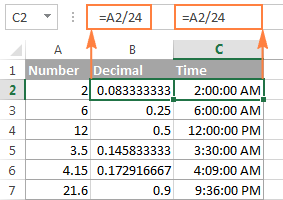That's all for today. If someone wants to get the first-hand experience with the formulas discussed in this article, you are most welcome to download the Sample workbook for converting time in Excel.

If you want to learn a few more helpful formulas to add and subtract times, calculate elapsed time and time difference, please check out other parts of our Excel Times tutorial (the links are available at the end of this page). I thank you for reading and hope to see you again next week.

### 212 responses to "How to convert time to decimal number, hours, minutes or seconds in Excel"

1.Vivek Wadhawan says:

Hi Svetlana,

I have a question,

How can I get the difference between "1/29/2019 9:48:13 AM" and "1/27/2019 12:06:36 PM" in "HH:MM:SS" format, what formula I should use for such cases ?

•Bob says:

Same question here. How do you convert DD:HH:MM:SS into HH:MM:SS ?

•Svetlana Cheusheva (Ablebits.com Team) says:

Hi Guys,

Sorry, I have overlooked this question somehow. To display the difference between the two dates in hours, minutes and seconds, use this format: [h]:mm:ss

The hour unit code enclosed in square brackets is used to display more than 24 hours. For more information, please see How to show over 24 hours, 60 minutes and 60 seconds in Excel.

2.onyeka says:

how can i convert an excel data of this format 25 mins to time data

3.onyeka says:

25mins,1 hr 8 mins convert to time

4.Jack says:

Hi,
Input: 0 13:15:20
Input format: d hh:nn:ss
D=days
h=hours
n=minutes
s=seconds
Output required: eg: 0.5523
A decimal value of the duration.
Thank you for any help.

5.Kevin says:

Hello,
I am creating a sheet to calculate how much time it will take to perform a series of daily tasks. I have a set number of items that require 7 minutes of work per item. For instance, on Monday, there are 24 items that take 7 minutes each, for a total of 168 minutes. I need a formula that shows how many hours and minutes it will take. I can do the math myself and know that it takes 2 hours and 48 minutes (2 hours=120 minutes, plus 48 minutes). How do I get this to work in Excel?

6.Brock says:

I am trying to calculate lap splits for track athletes. I would like to calculate this in minutes, seconds and hundredths of a second. If I have elapsed time entered into a spreadsheet. Is there a formula that would calculate lap splits?

7.Alex says:

Hi,
Is there a way to convert from 1 to 6 digits in a column to time HH:MM:SS
110759 11:07:59
110739 11:07:39
110723 11:07:23
41727 04:17:27
41714 04:17:14
124 00:01:24
106 00:01:06
48 00:00:48
24 00:00:24
I use the Text to column, fixed width function but , when they are not the same number of digits in a row they do not split properly.
Tried the Custom format by adding 0 in front of the digits and it shows 6 digits in each cell but when I try to use the Text to column it ignores the format.
Any ideas?

•Bob says:

this is exactly what i'm searching for help on too. would love to see if anyone knows of a way to do this.

•Sridhara B says:

Try with Delimited & space

8.Shaun says:

I have time format in 1 days 3 hours 24 minutes.
I need to change this to actual hours and minutes.

Any help much appreciated.
Thanks

9.Shaun says:

This is a small sample of my data that I need to convert to [h]:mm.
I can remove the words days, hrs and mins, although it's sort of a manual process by replacing them with nothing.
I will be adding it all up and providing some statistical info on the changes, as there are two columns with similar data. One for before and another for after. Sometimes the second column has no value, contains the word "Removed" or is Zero "0 mins".
1 days 7 hrs 49 mins
6 hrs 10 mins
14 hrs 25 mins
8 hrs 50 mins
18 hrs 4 mins
17 hrs 58 mins
1 hrs 21 mins
4 days 2 hrs 34 mins
2 days 20 mins
I need help. I found something similar, but not quite the same. Also it's been written in a function. I know I have to call a function, but am not totally sure how this would be done.
Thanks for any assistance or if someone can write a macro or VBA code for me?
Much appreciated.

10.Pavan says:

Dear
Our worker overtime in number(6.83) we want change in hour pls solve with example

•RJ says:

6.83 multiplied by 24 then custom format and select hh:mm

•RJ says:

My fault should be divided by 24 then custom format and select hh:mm

11.RAKESH says:

HELLO DEAR,
CAN I CONVERT NUMBER FORMET FOR EXAMPLE 1.75 IN TO TIME FORMET FOR EXAMPLE 1.45 HOURS IN MS EXCEL.
9015223435

•Navish says:

I UNDERSTAND THAT YOU WOULD LIKE TO CONVERT NUMBER FORMAT (FOR EXAMPLE 1.75 IN TO TIME FORMAT WHICH IS 1.45 HOURS) IN MS EXCEL.

> FIRSTLY, CONSIDER 1.75(WHICH IS IN GENERAL FORMAT) IN CELL A1. USE THE FORMULA IN CELL A2 AS =A1/24(THIS IS LIKE YOU ARE CONVERTING IT INTO HOURS). YOU WILL GET THE VALUE 0.072916667(WHICH IS GENERAL FORMAT).
FINALLY, SELECT THE CELL A2 AND CONVERT INTO TIME FORMAT('HOME' TAB > GENERAL TO 'TIME'), YOU WILL GET THE VALUE 01:45:00.

•Emily says:

Navish, is there a way to do it the other way? If I have a time (let's say 8:30), how do I convert it to 8.5 in number format in excel?

Thank you!

12.Ville says:

Hello,
Is there a format type or other way to quickly type hours to cell?
For example I want to add time 21:00 by typing only "21".
I have tried multiple different formats and if I type "21" it turns out 0:00.
Any idea about the format I should use?

•Davor says:

Ville,
Format cell as Custom, adding in "Type:" 00":"00
So for eg. 7:15 you type 715

Hope this helps,
Davor

13.Srikanth says:

How to convert number 102 to HH:MM

14.Nor Hashim says:

Thank you, Svetlana! For weeks, I have been looking all over for help that covers all my need for time/date conversion. Your answers work perfectly with my data!

15.AJen says:

Thanks for the tutorial and for answering questions. I'm trying to convert a cell that is 'x days, y hours, z minutes, and t seconds' into minutes. I've tried a few of the recommended solutions however none have worked. What might I be missing or have wrong (i.e critical spacing I'm adding/missing or a specific format I need to have in the answer cell)? Also note, sometimes days, hours, , minutes, and/or seconds might have a 0 value. Often when I type them in the first referenced cell is highlighted like normal but every referenced cell following is not highlighted. Thanks in advance for your help. Here are the suggested formulas I've tried:
=INT(LEFT(C8 ;FIND("days"; C8) - 1)) * 24 * 60 + INT(MID(C8 ; FIND(" "; C8) + 1; FIND("hours"; RIGHT(C8 ; LEN(C8) - FIND(" "; C8))) - 1)) * 60 + INT(MID(C8 ;FIND(" "; C8;FIND("hours"; C8))+1;FIND("minutes"; C8)-FIND(" "; C8 ;FIND("hours"; C8)) - 1))

And I've tried this one:
=IF(ISERROR(FIND("day", A1)), 0, INT(TRIM(LEFT(A1, FIND("day", A1) -1))) * 24 * 60) + IF(ISERROR(FIND("hour", A1)), 0, INT(MID(A1,IF(FIND("hour",A1)-6<0,1,FIND(" ",A1,FIND("hour",A1)-6)),FIND("hour",A1)-IF(FIND("hour",A1)-6<0,1,FIND(" ",A1,FIND("hour",A1)-6)))) * 60) + IF(ISERROR(FIND("min", A1)), 0, INT(MID(A1, FIND(" ", A1, FIND("min", A1)-6), FIND("min", A1)-FIND(" ", A1, FIND("min", A1)-5))))

16.Sam says:

27days * 10:30hours = ans in hours
How to formula for this type of sums ?

17.Sam says:

25days * 10:30 hour = ? In hh:mm format ?
Whts the formula like this calculation

18.Gagneet says:

Need help converting hh:m to Degrees on a circle. For example 2:53 to degrees.

19.Gagneet says:

Would it be converting 2:53 to Hours then multiplying by 30 degrees?
2:53*24=2.8833 then 2.8833*30=86.50 Degrees

20.RAJESH LAUL says:

THANKS AND REGARDS FOR SUPPORT

21.Swap says:

I have to convert value excel calculation is 100 qty is one hour this value i have convert in time format

22.endy says:

Hi all,

how to split 1 day 3 hours 17 minutes into hour and minutes separately?

23.Khatijah Nasir says:

How to format hours by using IF...
Let's say I want from 06:00am to 22:00pm be "morning" and 22:00pm to 06:00am be "night".
Thank you.

•Michael says:

Creating an Excel spreadsheet to use as a time card to keep track of the hours worked, I have used the formula =IF(end>start, end-start, 1-start+end) to reflect there are times I'll elapse (i.e. - go beyond midnight) to calculate the total number of hours. Now I need to convert that time into an hour and decimal minutes format to be able to calculate regular pay and overtime pay. How do I do that?

24.GIREESH KUMAR says:

I WANT TO CONVERT HOURS TO minutes
A/S CAST OFF TOTAL HRS TOTAL minutes
15:50 19:25 3:35

25.Jitin Kaushik says:

How can convert 1 productivity = 8:30 hours in excel sheet example .5 = 4:15hours and .25 = 2 hours
Time difference 15,30,45,60 minutes

26.Shahid says:

Please support how to convert time into hours duration i.e. OUT Time 10:23:28 AM and IN Time 7:27:31 PM need to calculate the duration in Excel for bulk data.

Thanks.

•Mary Trifuntova (Ablebits.com Team) says:

Hello Shahid,
If you need the time difference in hh:mm:ss format, here is the formula for you:
=IF(A2<A1, A2+1, A2)-A1

Supposing the IN time is in A1 and OUT - in A2. If however, you need it to be a just number, the following formula will do the job:
=HOUR(IF(A2<A1, A2+1, A2)-A1)+MINUTE(IF(A2<A1, A2+1, A2)-A1)/60

27.Dilip says:

Sir,

Date Shift ArrTim LateHrs DepTim EarlHrs WrkHrs OvTim Present

01/12/2019 G 0.00
02/12/2019 G 9.56 18.00 8.04 1.00
03/12/2019 G 9.56 18.01 8.05 1.00
04/12/2019 G 9.46 18.01 8.15 1.00
05/12/2019 G 9.57 18.01 8.04 1.00
06/12/2019 G 9.47 18.18 8.31 1.00
07/12/2019 G 9.57 18.00 8.03 1.00
08/12/2019 G 0.00
09/12/2019 G 9.47 18.00 8.13 1.00
10/12/2019 G 9.56 18.01 8.05 1.00
11/12/2019 G 9.57 18.00 8.03 1.00
12/12/2019 G 9.47 18.00 8.13 1.00
13/12/2019 G 9.58 18.01 8.03 1.00
14/12/2019 G 10.26 0.26 18.00 7.34 1.00
15/12/2019 G 0.00
16/12/2019 G 10.05 0.05 18.01 7.56 1.00
17/12/2019 G 9.56 18.00 8.04 1.00
18/12/2019 G 9.56 18.00 8.04 1.00
19/12/2019 G 9.57 18.00 8.03 1.00
20/12/2019 G 9.57 18.01 8.04 1.00
21/12/2019 G 9.58 18.00 8.02 1.00
22/12/2019 G 0.00
23/12/2019 G 9.56 18.00 8.04 1.00
24/12/2019 G 9.57 18.00 8.03 1.00
25/12/2019 G 9.57 18.00 8.03 1.00
26/12/2019 G 9.55 18.00 8.05 1.00
27/12/2019 G 10.06 0.06 18.00 7.54 1.00
28/12/2019 G 9.53 18.00 8.07 1.00
29/12/2019 G 0.00
30/12/2019 G 9.58 18.00 8.02 1.00
31/12/2019 G 9.58 17.09 0.51 7.11 1.00

kindly solve this how we calculated the total working hour's in that sheet
here is mentioned that in time and out time and difference how many hour's working an employee in our office and after how to calculate total working hour's in a month kindly reply

Thanks.

28.SUKHVINDER KAUR says:

I WANT TO ADD TIME VALUE AS U/M:-
A1 = 93:01:00
B1 =13349:50:00
C1 = REQUIRED FORMULA TO ADD ABOVE MENTIONED TIME VALUE.
THANKS

29.Michael says:

Creating an Excel spreadsheet to use as a time card to keep track of the hours worked, I have used the formula =IF(end>start, end-start, 1-start+end) to reflect there are times I'll elapse (i.e. - go beyond midnight) to calculate the total number of hours. Now I need to convert that time into an hour and decimal minutes format to be able to calculate regular pay and overtime pay. How do I do that?

30.Brian says:

Trying to convert to below to minutes with out text

Notify to Last Assign Transit Time Total In Progress Time Turnaround Time
1h 14m 1m 40m 58m

31.Hastings Wauka Mwale says:

Start 6:45 7:28 7:00 7:27 6:28 6:59 13:00
Finish 22:48 23:00 23:35 22:15 22:54 22:31 22:03
H:min 16:03 15:32 16:35 14:48 16:26 15:32 9:03
How do we add these hours and mins to hours only in Excel?

32.Chai Yang says:

hour calculation - after sum total hour is 18:90: I want to I change from the 18:90 (18 hrs 90 min) in order to become 19 hrs 30 min. Can you I change it in Excel?

thank you

33.Sebastian says:

Hello everybody!
I can't find within the answers … I'm having difficulties with changing minutes and second in format 35:12 into seconds. Result I am looking for should be 2112.
Thanks"

34.akashsaini says:

how to convert in date & Time format below value
20001124204143.0Z

•akashsaini says:

I will try with Mod formula but 2020 value showing wrong this will shows in filter as 2020 Jan to Dec but still running feb month and when we check in Ctrl+Shift+3 format in 2020 date then showing another date.

35.Naresh says:

If Hour minute and second value 1370:06:09 in this format then how to separate hours minute and second.
Pls suggest.

36.JERRY says:

11.2hrs convert to days,hrs,min
formula pls?

37.Dave Chladek says:

I know I'm asking a lot here but could you explain how to convert "Duration in seconds (Unix)" to a readable format of years,months,weeks,days,seconds in Excel? I see all sorts of ways to convert date to date, etc but not "duration" of time in seconds! I need a way to convert it into a readable format of years, months, weeks, days, hours and seconds. I can do it in Perl, C and others but not in Excell. If you can help I have a few examples of what the end result needs to be in specific representations such as (11W) for 11 weeks or (13W2D) for 13 weeks and two days or (1Y26W6D) 1 year, 26weeks, 6days and even further. Here are a few examples...

Duration in Seconds:
1) 6652800 and the output would be 11W or 11(W)eeks
2) 8035200 and the output would be 13W2D or 13(W)eeks 2(D)ays
3) 47779200 and output would be 1Y26W6D or 1(Y)ear 26(W)eeks and 6(D)ays
4) 47779200 seconds and output would be 1Y6M5D3H16M51S or 1(Y)ear,6(M)onths,5(D)ays,3(H)ours,16(M)inutes,51(S)econds
and/or convert years into months...
5) 47779200 output to 18M5D3H16M51S or 18(M)onths,5(D)ays,3(Hours),16(M)inutes,51(S)econds

Does this make sense? I'm not in a big hurry so if this is to much at this time don't worry about it. I'll keep looking around and I want to say: I really appreciate your taking the time to help with your explanations and this web site, these examples and explanations some of my make daily stuff much easier and I really appreciate how you offer so much expertise to everyone.

Many Thanks!!
Dave C.

38.Maty says:

Has anyone actually found a resolution conversion for "D HRS:MIN:SEC" to "HRS"?
eg. 1 22:12:00

39.Danielle Parizeau says:

Hello,
In my excel spreadsheet I have column totally the number of hours in total (ex: from (9 am to to 10:30 am = 1.3) I would like to convert the 1.3 to 1.5. How add this function in my existing formula: =IF(k3≥J3,K3-J3,1-K3+J3)*L3..... L3 being the hours in total.
Thank you!

•Alexander Trifuntov (Ablebits.com Team) says:

Hello Danielle!
If I understand your task correctly, you need to convert time from HH:MM:SS format to decimals. If so, you just need to multiply it by 24 (for example, =А3*24) and set the General format for this cell.

40.Geoff says:

I've downloaded a csv file that has a time on it but when I convert to Excel and use a formula on it it shows as the decimal equivalent of the number and not the time ,I cannot get to change to a time format that equals the original downloaded number/time

•Alexander Trifuntov (Ablebits.com Team) says:

Hello Geoff!
Please try the formual below to convert time from a decimal number to "hh:mm:ss":
=TIME(D1, MOD(D1, 1)*60, 0)

That should do the trick.

•Geoff says:

thank you but the issue I have would be say i wanted to concatenate 2 cells ..... one with contents CPark and the other 10:46 AM the concatenate function results CPark0.4486111111111111 rather than CPark10:46
your suggestions effect upon the original 10:46 AM cell returns 12:26AM
thank you for your help so far though

•Alexander Trifuntov (Ablebits.com Team) says:

Hello Geoff,

Thank you for the clarification. In this case, formual below will convert your data from time to text:
=CONCATENATE(A1, TEXT(B1, "hh:mm AM/PM"))

That should do the trick.

•geoff says:

sorry that is not the answer thanks Alexander although its probably on the right track
I cannot find the solution anywhere on the internet

•geoff says:

working now thank you Alexander !!!!

•Geoff says:

so when I use a look up to cross reference 2 sheets in the same workbook even though I have the concatenate correct it doesn't pick up the cell I need ...can anyone have a look at the file if I send it to them to see where Im going wrong please ? I'm sure its due to the time format mentioned above that even though they look the same in the cell ref they are not .....

41.Santiago Grullon says:

How do you convert 06:55 into 0655 in Excel. I want to to eliminate the colon.
Thank you.

•Alexander Trifuntov (Ablebits.com Team) says:

Hello Santiago!
You can convert time to text.

=TEXT(A1,"[hh]mm")

42.Yudi says:

Hello dear..
I am from Indonesia..
How to stop auto convert number from general to custom
ex.
from 1. auto convert to 01.00
from 10. auto convert to 10.00
from 100. auto convert to 100.00.00

43.Erica says:

I would like to convert time to a numerical number to add up total hours.
Example:
6:00AM-2:00PM=8 hours

•Alexander Trifuntov (Ablebits.com Team) says:

Hello Erica!
Specify what you want to calculate. Your formula counts the time from 2 PM to 6 AM. And this is not 8, but 16 hours. If you want to calculate the time from 6 AM to 2 PM, then the formula is necessary
= 2: 00 PM-6: 00AM
To convert a result from time to number, use the formula
= (2:00 PM-6:00AM) * 24
And set the number format in this cell

44.libbrecht says:

Hi,
How to convert 9,30 in decimal 9,50 or 8,45 in 8,75.
The times I receive in Excel are the effective times marked with a comma (none :)
tnx

•Alexander Trifuntov (Ablebits.com Team) says:

Hello!
The rounding of numbers in your examples occurs according to different rules. Therefore, I can not offer you a general formula for your two tasks. I recommend paying attention to the MROUND function. For your first example, the formula is suitable

=MROUND(A1,0.5)

45.Shelley says:

Hello;
When converting a number under 24 hours the formula number/24 works but if totalling hours over a week, the formula does not work if the total goes over 1 day or 24 hours.
Thank you

•Shelley says:

I am looking for the excel formula please :0)

•Alexander Trifuntov (Ablebits.com Team) says:

Hello!
To be able to show more than 24 hours in a cell, please use this time format: "37:30:55". You can find it in the list of Excel time formats.

46.deepak says:

i have 261 minute. needs to be convert as HH:MM

•Alexander Trifuntov (Ablebits.com Team) says:

Hello!
The formula below will do the trick for you:

=CONVERT(D1,"mn","hr")/24

Set the cell time format hh:mm

47.Sudhakar says:

2 days 19 hours 40 minutes

Its convert to 67.40 Dec... Can u plz send the excel formula

•Alexander Trifuntov (Ablebits.com Team) says:

Hello!
What does 67.4 mean? What are these units of measurement?
2 * 24 + 19 + 40/100? This number doesn't make sense.

48.Anees says:

August 1, 2020 3:52:15 PM AST
how to change this one as date format , tried a lot, can you please guide me.

49.Youssef Ouazzani touhami says:

bonsoir,
j'ai un petit problème sur excel, c'est que j'ai besoin de créer des colonnes avec ces donnée

- column 1: Julianne day (1 to 365)
- column 2: Solar time in decimal format (00:00:00) with interval of 5 min (from 1 to 12 for each hour))

mon problème c'est que j'arrive pas a trouver une formule pour gérer l'intervalle de 5min sur un ans.

merci

•Alexander Trifuntov (Ablebits.com Team) says:

Hello!
I am not sure I fully understand what you mean. Give an example of the source data and the expected result.
It’ll help me understand it better and find a solution for you.

50.Sheldon Zaccanti says:

am try to convert actual minutes to decimals such as 4.11 hours worked to equal 4.18 hours and 3.32 hours to 3.53 and so forth so it works like a time clock where 15 minutes =.25 -30 min =.5 45 minutes =.75 thank you in advance